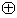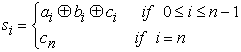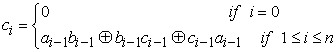﻿ Design of Digital Multiplier with Reversible Logic by Using the Ancient Indian Vedic Mathematics Suitable for Use in Hardware of Cryptosystems

### Design of Digital Multiplier with Reversible Logic by Using the Ancient Indian Vedic Mathematics Sui...

Giridhari Muduli, Siddharth Kumar Dash, Bibhu Datta Pradhan, Manas Ranjan JenaOPEN ACCESSPEER-REVIEWED

## Design of Digital Multiplier with Reversible Logic by Using the Ancient Indian Vedic Mathematics Suitable for Use in Hardware of Cryptosystems

Giridhari Muduli1, Siddharth Kumar Dash1, Bibhu Datta Pradhan1, Manas Ranjan Jena1,1Department of ETC, SIET, Dhenkanal, Odisha, India

### Abstract

Differential Power Analysis (DPA) presents a major challenge to mathematically secure cryptographic protocols. Attacks can break the encryption by measuring the energy consumed in the working digital circuit. To prevent this types of attack, this paper proposes the use of reversible logic for designing a high speed complex multiplier (ASIC) based on Vedic mathematics in cryptosystem. Reversible logic is gaining significance in the context of emerging technology such as quantum computing. Ideally, reversible circuits do not loose information during computation. Thus, it would be of great significance to apply reversible logic to design for secure cryptosystem. The idea for designing the multiplier unit is adopted from ancient Indian mathematics “Vedas”. On account of these formulas, the partial products and sums are generated in one step which reduces the carry propagation from LSB to MSB. The implementation of the vedic mathematics & their application to the complex multiplier ensure substantial reduction of propagation delay in comparison with DA based architecture (distributed arithmetic) & parallel adder based implementation which are commonly used. The functionality of these circuits was checked & performance parameters like propagation delay and dynamic power consumption were calculated by spice specter using standard 90nm cmos technology.

### At a glance: Figures

123
Prev Next

• Muduli, Giridhari, et al. "Design of Digital Multiplier with Reversible Logic by Using the Ancient Indian Vedic Mathematics Suitable for Use in Hardware of Cryptosystems." International Transaction of Electrical and Computer Engineers System 2.4 (2014): 114-119.
• Muduli, G. , Dash, S. K. , Pradhan, B. D. , & Jena, M. R. (2014). Design of Digital Multiplier with Reversible Logic by Using the Ancient Indian Vedic Mathematics Suitable for Use in Hardware of Cryptosystems. International Transaction of Electrical and Computer Engineers System, 2(4), 114-119.
• Muduli, Giridhari, Siddharth Kumar Dash, Bibhu Datta Pradhan, and Manas Ranjan Jena. "Design of Digital Multiplier with Reversible Logic by Using the Ancient Indian Vedic Mathematics Suitable for Use in Hardware of Cryptosystems." International Transaction of Electrical and Computer Engineers System 2, no. 4 (2014): 114-119.

 Import into BibTeX Import into EndNote Import into RefMan Import into RefWorks

### 1. Introduction

Side Channel attacks against cryptographic systems exploit physical characteristics of a device, rather than direct code breaking methods. One such technique is Differential Power Analysis (DPA), which uses the power consumption of a cryptographic device such as a smart card. It is known that the amount of power consumed by the device varies depending on the data and the instruction performed during different parts of an algorithm’s execution, thus an attacker directly observes a device’s power consumption by simply examining the power traces. It is possible to determine the characteristics of a cryptographic device and the key of the cryptographic algorithm being used. In this work, we proposes the use of reversible logic to thwart attacks against cryptographically secure hardware based on DPA. Researchers have shown that for reversible logic computation, each bit of lost information generates kTln2 joules of heat energy, where k is Boltzmann’s constant and T is the absolute temperature at which the computation is performed. Reversible circuit does not loose information and thus kTln2 joules of heat energy will not be dissipated. Furthermore, voltage – coded logic signal s have an energy of Esig =1/2cv2, and this energy is dissipated whenever the node voltage changes in the irreversible CMOS technology. It is estimated that reversible logic also helps to save energy by using charge recovery . Younnis has fabricated an 8x8 reversible multiplier array using SCRL gates and measured an energy saving of over 99% conventional CMOS implementations of the same circuits. Thus, the application of reversible logic to the field of hardware cryptography is proposed here to guard against DPA attack, as, ideally no energy will be dissipated in the reversible circuits. Addition and modulo multiplication are the two major power hungry operations in the ALU of a crypto-procssor .

A quantum computer will be viewed as a quantum network (or a family of quantum networks) composed of quantum logic gates; each gate performing an elementary unitary operation on one, two or more two state quantum systems called qubits. Each qubit represents an elementary unit of information; corresponding to the classical bit values 0 and 1.Any unitary operation is reversible and hence quantum networks must be built from reversible logic components. Quantum computers of many qubits are extremely difficult to realize thus the number of qubits in the quantum circuits needs to be minimized. This sets the major objective of optimizing the number of ancilla input qubits and the number of the garbage outputs in the reversible logic based quantum circuits. The constant input in the reversible quantum circuit is called the ancilla input qubit (ancilla input bit),while the garbage output refers to the output which exists in the circuit just to maintain one-to-one mapping but is neither one of the primary inputs nor a useful output. Thus, the inputs regenerated at the outputs are not considered as garbage outputs .

Multiplier has immense importance in Digital Signal processing (DSP) and Image processing (IP). To implement the hardware module of Discrete Fourier transform (DFT), Discrete Sine Transformation (DST) and modern broadband communications.

In algorithmic and structural levels, a lot of multiplication techniques had been developed to enhance the efficiency of the multiplier; which encounters the reduction of the partial products and/or the methods for their partial products addition, but the principle behind multiplication was same in all cases. Vedic mathematics is the ancient system of Indian mathematics which has a unique technique of calculations based on 16 sutras (formulae). “Urdha-tiryakbyam” is a Sanskrit word means vertically and crosswise formula used for multiplication. In this work we formulate this mathematics design for multiplier and again it is implemented with the reversible logic for the power loss minimization in cryptosystem which will be very efficient against crypto-analysis attack .

### 2. Proposed Multiplier Architecture

The conventional mathematics is an integral part of engineering education as most engineering system designs are based on various mathematical approaches. A multiplier is one of the key hardware blocks in most digital signal processing systems. With advances in technology, many researchers have tried to design multipliers which offer either of the following- high speed, low power consumption, regularity of layout and hence less area or even combination of them in multiplier. We appreciate the efforts put by Jagadguru Swami Sri Bharati Krishna Tirthaji Maharaja to introduce Vedic Mathematics and also acknowledge the work of various people regarding Vedic Mathematics as the Vedic mathematics approach is totally different and considered very close to the way a human mind works. The multiplication of numbers is utilized in almost all branches of engineering; therefore the demand for high efficiency multiplier architecture increases. In this paper for the proposed digital multiplier we have used Urdhva-Tiryagbhyam sutra of Vedic mathematics. Vedic mathematics is based on sixteen sutras which serve as somewhat cryptic instructions for dealing with different mathematical problems The basic rule for the multiplication of two numbers of 4 digit is shown using the line drawing as follows .

Urdhva-Tiryagbhyam

Urdhva-Tiryagbhyam is the general formula applicable to all cases of multiplication and also in the division of a large number by another large number. It multiplies the numbers in the vertical and crosswise fashion so in English it is named as vertically and crosswise algorithm .

2.1. Comparison between Normalmethod of Multiplication and Vedic Mathematics Multiplication

#### Table 1. Comparision between normal method multiplication & vedic mathematics multiplicationDownload asPowerPoint Slide

Veiw figureView current table in a new window

2.2. Multiplier Implementation

In this paper, the proposed multiplier architecture is implemented in VHDL (Very High Speed Integrated Circuited Hardware Description Language) and the FPGA synthesis is done using Xilinx ISE 8.2i. The design is optimized for speed and area using Xilinx, device family: Virtex XC4VLX15, package SF363,speed grade-12. The Xilinx Virtex XC4VLX15-12 device is to be applied and the device contains 6144 slices and 12288 input Look Up Tables and 240 bonded Input/output pads.

The implementation style is the Fully partitioned Vedic multiplier. Here for 8*8 multiplier implementation, 4x4bits multiplier units have been used as components. Then the reversible ripple carry adders are implemented for addition need. Finally, the result of the multiplier is displayed through adequate size of output port .

We present the design of reversible ripple carry adder with no input carry(c0) and is designed without any ancilla inputs and the garbage outputs. The proposed method improves the quantum cost and the delay of the reversible ripple carry adder compared to the existing design approaches which have optimized the adder design in terms of number of ancilla inputs. Consider the addition of two n bit numbers ai and bi stored at memory locations Ai and Bi, respectively, where 0 ≤ i ≤ n−1. Further, consider that memory location An is initialized with z ∈ {0, 1}. At the end of the computation, the memory location Bi will have si, while the location Ai keeps the value ai. The additional location An that initially stores the value z will have the value zsn at the end of the computation. Thus An will have the value of sn when z=0. Here, si is the sum bit produced and is defined as:where ci is the carry bit and is defined as:The proposed design methodology of generating the reversible ripple carry adder with no input carry minimizes the garbage outputs by producing the carry bits ci based on the inputs ai-1, b i-1 and the carry bit c i-1 from the previous stage. Once all the carry bits ci are generated they are stored at memory location A i-1 which was initially used for storing the input a i-1 for 0 ≤ i ≤ n − 1. After the generated carry bits are used for further computation, the location Ai are restored to the value ai while the location Bi stores the sum bit si for 0 ≤ i ≤ n − 1. Thus restoring of location Ai to the value ai helps in minimizing the garbage outputs. Since no constant input having the value as 0 is needed in the proposed approach, it saves the ancilla inputs. The proposed methodology of generating the reversible ripple adder circuit without input carry is referred as methodology 1 in this work. The proposed methodology is generic in nature and can design the reversible ripple carry adder circuit with no input carry of any size. The steps involved in the proposed methodology is explained for addition of two n bit numbers ai and bi, where 0 ≤ i ≤ n−1. An illustrative example of generation of reversible ripple carry adder circuit that can perform the addition of two 8 bit numbers a=a0:::a7 and b=b0:::b7 is also shown.

2.3. Steps of Methodlogy (Reversible Adder Circuits with no Input Carry)

(1) For i=1 to n-1:

At pair of locations Ai and Bi apply the CNOT gate such that the location Ai will maintain the same value, while location Bi transforms to (*Ai*Bi), where *Ai and *Bi represent the values stored at location Ai and Bi. The step 1 is shown for reversible ripple carry adder circuit that can perform the addition of two 8 bit numbers in Figure 2.5(a).

(2) For i=n-1 to 1:

At pair of locations Ai and Ai+1 apply the CNOT gate such that the location Ai will maintain the same value, while the location Ai+1 transforms to (*Ai*Ai+1). The step 2 is shown for reversible 8 bit adder circuit in Figure 2.5(b).

(3) For i=0 to n-2:

At locations Bi, Ai and Ai+1 apply the Toffoli gate such that Bi, Ai and and Ai+1 are passed to the inputs A, B, C, respectively, of the Toffoli gate. The step 3 is shown for reversible 8 bit adder circuit in Figure 2.5(c).

(4) For i=n-1 to 0:

At locations Ai, Bi and and Ai+1 apply the Peres gate such that Ai, Bi and and Ai+1 are passed to the inputs A, B, C, respectively, of the Peres gate. The step 4 is shown for reversible 8 bit adder circuit in Figure 2.6(a).

(5) For i=1 to n-2:

At pair of locations Ai and Ai+1 apply the CNOT gate such that the location Ai will maintain the same value, while location Bi transforms to the value (*Ai*Bi). The step 5 is shown for reversible 8 bit adder circuit in Figure 2.6(b).

(6) For i=1 to n-1:

At pair of locations Bi and Ai apply the CNOT gate such that the location Ai will maintain the same value, while location Bi transforms to the value (*Ai*bi). This final step will result in a reversible adder circuit that can perform the addition of two n bit numbers. For reversible 8 bit adder circuit, the design is shown in Figure 2.6 (c).

### 3. Simulation Results & Analysis

The synthesis & simulation is performed by using softwares like Xilinx ISE 8.2i. which is further used for coding, testing and simulation of VHDL programs. The design is optimized for speed and area using Xilinx, device family: Virtex XC4VLX15, package SF363,speed grade-12.

### 4. Conclusion

This paper proposes the novel idea of applying reversible logic on a complex multiplier. This design is based on the formulas of the ancient Indian Vedic Mathematics, highly suitable for use in hardware of cryptosystems making secure against DPA attacks. It is also having future scope in wide application in VLSI Signal Processing.

To design a multiplier which could be used for Cryptographic purposes this multiplier can be used in the field of different secure communications. In the future work of the multiplier most emphasis is dedicated towards power loss minimizations, time delay minimization of the multiplier. Moreover, the aim of future work is to make the multiplier a real time implementation in different circuits.

### Acknowledgement

The authors sincerely thank to the H.O.D & all the staff of Dept. of ETC, SIET, DHENKANAL,ODISHA for constant encouragement and support directly or indirectly.

### References

  Bayrakci, A. and Akkas, A.. “Reduced delay bcd adder”, Proc. Application -specic Systems, Architectures and Processors. 266-271, 2007.In article  Biswas, A. K., Hasan, M. M., Chowdhury, A. R., and Hasan Babu, H. M. “Efficient approaches for designing reversible binary coded decimal adders”, Microelectron. J. 39, 12, 1693-1703, 2008.In article CrossRef  Bruce, J. W., Thornton, M. A., Shivakumaraiah, L., Kokate, P. S., and Li, X. “Efficient adder circuits based on a conservative reversible logic gate”, Proc. IEEE Symposium on VLSI, 2002. 83-88, 2002.In article  Cuccaro, S. A., Draper, T. G., Kutin, S. A., and Moulton, D. P. “A new quantum ripple-carry addition circuit”, http://arXiv.org/quant-ph/0410184,2004.In article  Haghparast, M., Jassbi, S., Navi, K., and O.Hashemipour. “Design of a novel reversible multiplier circuit using hng gate in nanotechnology”, World App. Sci. J. 3, 6, 974-978, 2008.In article  Maslov, D. and Dueck, G. W. “Reversible cascades with minimal garbage”, IEEE Trans. Computer-Aided Design, 23, 11 (Nov.), 1497-1509, 2004.In article  Mohammadi, M., Eshghi, M., Haghparast, M., and Bahrololoom, A. “Design and optimization of reversible bcd adder/subtractor circuit for quantum and nanotechnology based systems”, World Applied Sciences Journal 4, 6, 787-792, 2008..In article  Shende, V. V., Prasad, A., Markov, I., and Hayes, J. “Synthesis of reversible logic Circuits”. IEEE Trans. on CAD 22, 710-722, 2003.In article CrossRef  S.K.Sastry, H.S.Shroff, Mahammad, S. N., and Kamakoti, V. “Efficient building blocks for reversible sequential circuit design”. Proc. the 49th IEEE Intl. l Midwest Symp.on Cir. and Sys. Puerto Rico, 437-441, 2006.In article  B. Parhami, “Computer architecture arithmetic algorithms & hardware architectures”, 2nd edition, Oxford university press, New York, 2010.In article  Anvesh kumar, Ashish raman,”Low power ALU design by ancient mathematics”, 978-1-4244-5586-7/10, 2010, IEEE.In article